# sklearn.model_selection.KFold¶

class sklearn.model_selection.KFold(n_splits=5, *, shuffle=False, random_state=None)[source]

K-Folds cross-validator

Provides train/test indices to split data in train/test sets. Split dataset into k consecutive folds (without shuffling by default).

Each fold is then used once as a validation while the k - 1 remaining folds form the training set.

Read more in the User Guide.

Parameters:
n_splitsint, default=5

Number of folds. Must be at least 2.

Changed in version 0.22: n_splits default value changed from 3 to 5.

shufflebool, default=False

Whether to shuffle the data before splitting into batches. Note that the samples within each split will not be shuffled.

random_stateint, RandomState instance or None, default=None

When shuffle is True, random_state affects the ordering of the indices, which controls the randomness of each fold. Otherwise, this parameter has no effect. Pass an int for reproducible output across multiple function calls. See Glossary.

StratifiedKFold

Takes class information into account to avoid building folds with imbalanced class distributions (for binary or multiclass classification tasks).

GroupKFold

K-fold iterator variant with non-overlapping groups.

RepeatedKFold

Repeats K-Fold n times.

Notes

The first n_samples % n_splits folds have size n_samples // n_splits + 1, other folds have size n_samples // n_splits, where n_samples is the number of samples.

Randomized CV splitters may return different results for each call of split. You can make the results identical by setting random_state to an integer.

Examples

>>> import numpy as np
>>> from sklearn.model_selection import KFold
>>> X = np.array([[1, 2], [3, 4], [1, 2], [3, 4]])
>>> y = np.array([1, 2, 3, 4])
>>> kf = KFold(n_splits=2)
>>> kf.get_n_splits(X)
2
>>> print(kf)
KFold(n_splits=2, random_state=None, shuffle=False)
>>> for train_index, test_index in kf.split(X):
...     print("TRAIN:", train_index, "TEST:", test_index)
...     X_train, X_test = X[train_index], X[test_index]
...     y_train, y_test = y[train_index], y[test_index]
TRAIN: [2 3] TEST: [0 1]
TRAIN: [0 1] TEST: [2 3]


Methods

 get_n_splits([X, y, groups]) Returns the number of splitting iterations in the cross-validator split(X[, y, groups]) Generate indices to split data into training and test set.
get_n_splits(X=None, y=None, groups=None)[source]

Returns the number of splitting iterations in the cross-validator

Parameters:
Xobject

Always ignored, exists for compatibility.

yobject

Always ignored, exists for compatibility.

groupsobject

Always ignored, exists for compatibility.

Returns:
n_splitsint

Returns the number of splitting iterations in the cross-validator.

split(X, y=None, groups=None)[source]

Generate indices to split data into training and test set.

Parameters:
Xarray-like of shape (n_samples, n_features)

Training data, where n_samples is the number of samples and n_features is the number of features.

yarray-like of shape (n_samples,), default=None

The target variable for supervised learning problems.

groupsarray-like of shape (n_samples,), default=None

Group labels for the samples used while splitting the dataset into train/test set.

Yields:
trainndarray

The training set indices for that split.

testndarray

The testing set indices for that split.

## Examples using sklearn.model_selection.KFold¶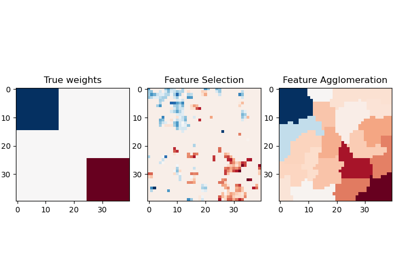Feature agglomeration vs. univariate selection

Feature agglomeration vs. univariate selection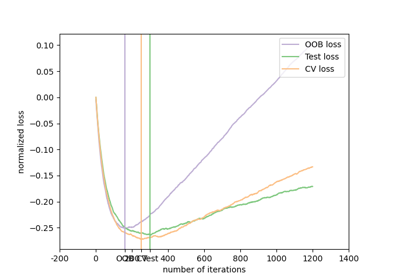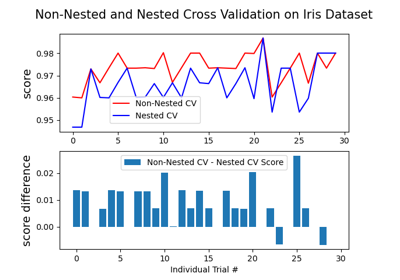Nested versus non-nested cross-validation

Nested versus non-nested cross-validation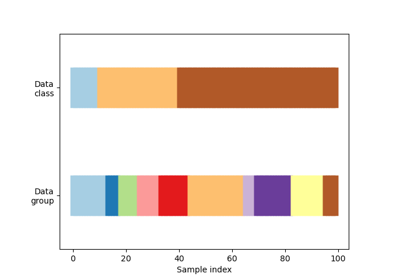Visualizing cross-validation behavior in scikit-learn

Visualizing cross-validation behavior in scikit-learn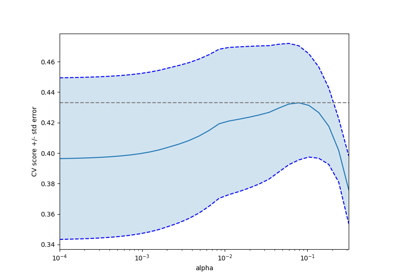Cross-validation on diabetes Dataset Exercise

Cross-validation on diabetes Dataset Exercise